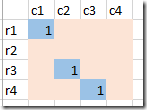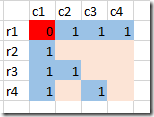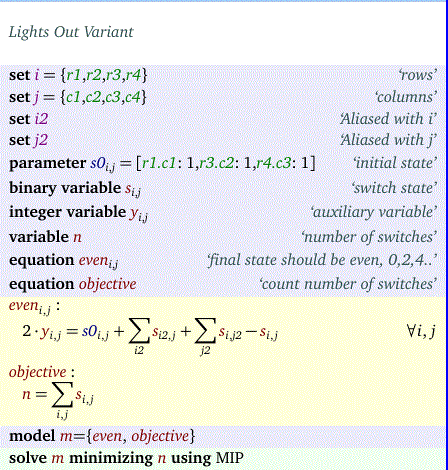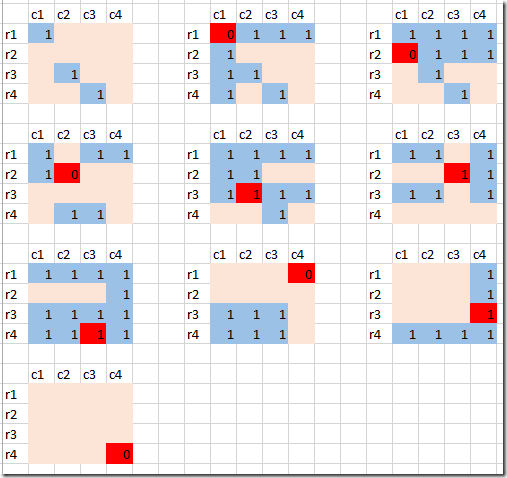Sunday, January 3, 2016

A variant of the Lights Out game

Suppose we have a grid like this, with some binary values turned on:This is our initial state s0. The goal is to turn all lights off, but there is a catch. When flipping a light switch all lamps in the same row and in the same column also flip. E.g. when we turn off light (1,1) we get:Which lights do we need to switch to make it dark? We want to minimize the number of needed switches. See this post.

Here is a MIP model:The idea is to add up the switches for each position (i,j) and then say: we want he final state to be even (i.e. 0,2,4,…). A simple way to require that a variable is even is to write it as 2*y where y is an integer variable. Interestingly, we are not interested in the value of y.

Note that we subtract s(i,j) because we double counted s(i,j) when adding up the switches in the same column and the same row.

The result is:

----     39 VARIABLE s.L  switch state

c1          c2          c3          c4

r1           1                                   1
r2           1           1           1
r3                       1                       1
r4                                   1           1

In the MIP model we apply these switches all at once, but if we do them one by one we would see:Now, a real difficult question is: what is the initial state s0 that requires the most switches?

Update

Hint: just adding s0 as variables and maximizing is not what I am after here (that would be easy but somewhat uninteresting). We want to find the initial configuration that requires the largest optimal switching plan. I.e. we end up with a bilevel mip. That is a notorious difficult thing.

More info on mixed-integer bilevel programming can be found in J.F.Bard, “Practical Bilevel Optimization: Algorithms and Applications”, 1999. Now on sale at Amazon for \$459!!